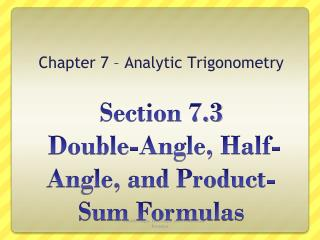DownloadDownload PresentationSection 7.3 Double-Angle, Half-Angle, and Product-Sum Formulas

Section 7.3 Double-Angle, Half-Angle, and Product-Sum Formulas

Download PresentationSection 7.3 Double-Angle, Half-Angle, and Product-Sum Formulas

- - - - - - - - - - - - - - - - - - - - - - - - - - - E N D - - - - - - - - - - - - - - - - - - - - - - - - - - -
Presentation Transcript

1. Chapter 7 – Analytic Trigonometry Section 7.3 Double-Angle, Half-Angle, and Product-Sum Formulas 7.3 - Double-Angle, Half-Angle, and Product-Sum Formulas

2. Double Angle Formulas • The double-angle formulas allow us to find the values of the trigonometric functions at 2x from their values at x. 7.3 - Double-Angle, Half-Angle, and Product-Sum Formulas

3. Proofs of Double Angle Formulas Now, you try the proof for cos2 and tan 2. 7.3 - Double-Angle, Half-Angle, and Product-Sum Formulas

4. Example • If and  lies in quadrant II, find the exact value of each of the following: 7.3 - Double-Angle, Half-Angle, and Product-Sum Formulas

5. Example • Find the exact value of 7.3 - Double-Angle, Half-Angle, and Product-Sum Formulas

6. Power Reducing Formulas • The power reducing formulas play an important part in calculus. 7.3 - Double-Angle, Half-Angle, and Product-Sum Formulas

7. Half Angle Formulas • NOTE: The choice of the + or – sign depends on the quadrant in which u/2 lies. 7.3 - Double-Angle, Half-Angle, and Product-Sum Formulas

8. Example • Find the exact value of cos105o. 7.3 - Double-Angle, Half-Angle, and Product-Sum Formulas

9. Example Verify the identity: 7.3 - Double-Angle, Half-Angle, and Product-Sum Formulas

10. Example Verify the identity: 7.3 - Double-Angle, Half-Angle, and Product-Sum Formulas

11. Product-to-Sum Formulas 7.3 - Double-Angle, Half-Angle, and Product-Sum Formulas

12. Examples – pg. 515 • Write the product as a sum. 7.3 - Double-Angle, Half-Angle, and Product-Sum Formulas

13. Sum-to-Product Formulas 7.3 - Double-Angle, Half-Angle, and Product-Sum Formulas

14. Examples – pg. 515 • Write the product as a sum. 7.3 - Double-Angle, Half-Angle, and Product-Sum Formulas

15. Example – pg. 515 • Prove the identity. 7.3 - Double-Angle, Half-Angle, and Product-Sum Formulas

16. Example • Write an equivalent expression for cos4x that does not contain powers of trigonometric functions greater than 1. 7.3 - Double-Angle, Half-Angle, and Product-Sum Formulas

17. More Examples • Prove the identity. 7.3 - Double-Angle, Half-Angle, and Product-Sum Formulas